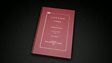Learn how to convert a denary number into a binary number using the divide by two method, with a worked example. If you keep dividing a denary number by two and noting down the remainders until you have nothing left, you’ll end up with a line of ones and zeroes. Flipping that number will give you the binary number.This clip is from:
Bitesize

This clip could be used as a whole class introduction to the topic of converting denary numbers to binary. Encourage students to try the process on their own, using other denary numbers. This could be used during a lesson to support students through independent learning. This would also be useful as part of revision on data representation for GCSE students.